Скачать презентацию On Capital Structure The impact of debt financing

ee95fc2f1e4b96679fd04dc2ee7d02e2.ppt

• Количество слайдов: 76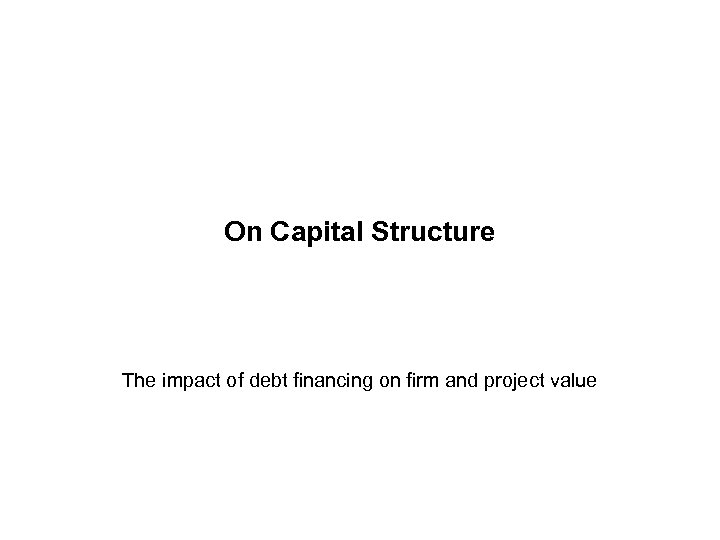On Capital Structure The impact of debt financing on firm and project value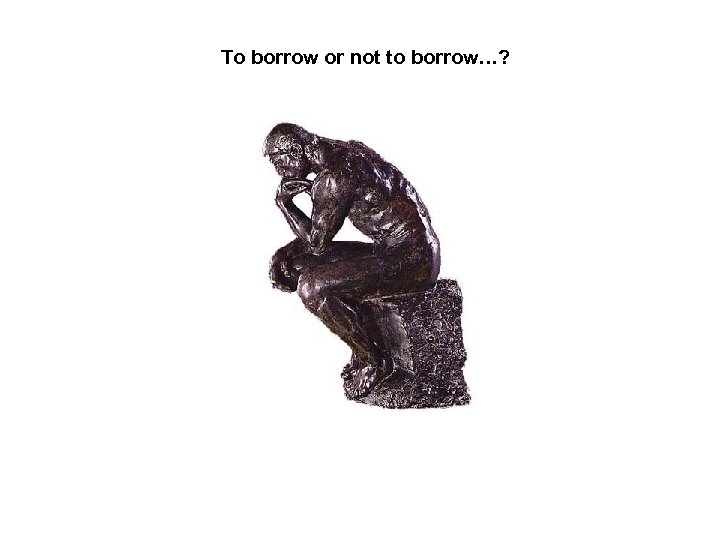To borrow or not to borrow…?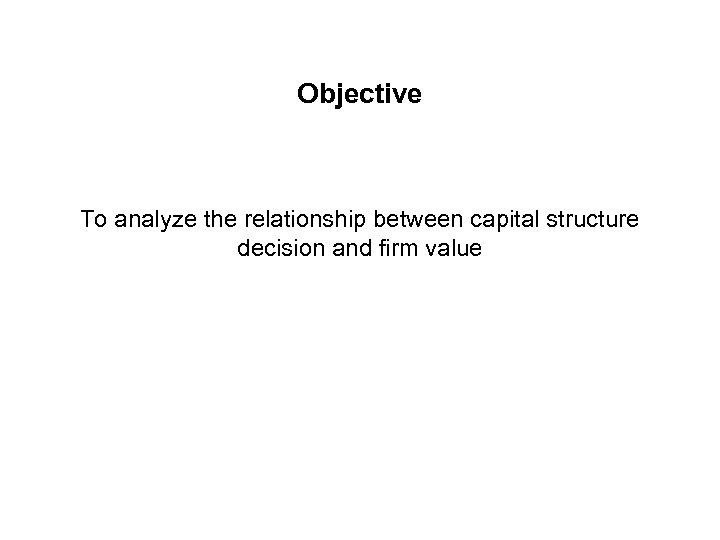Objective To analyze the relationship between capital structure decision and firm value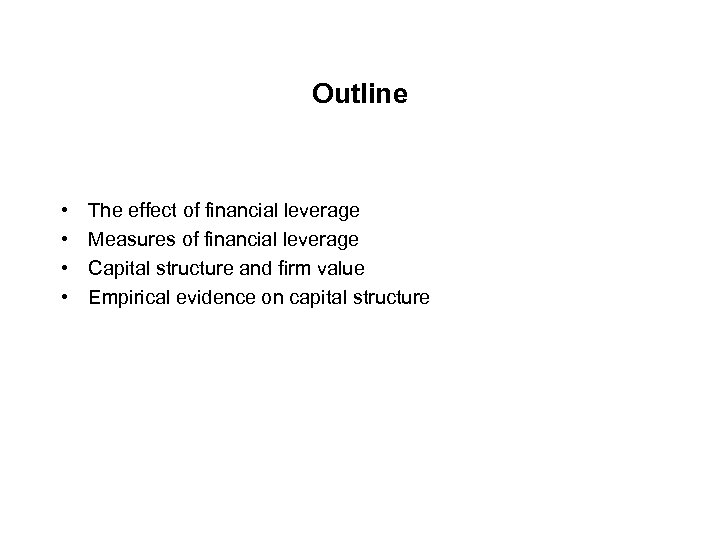Outline • • The effect of financial leverage Measures of financial leverage Capital structure and firm value Empirical evidence on capital structure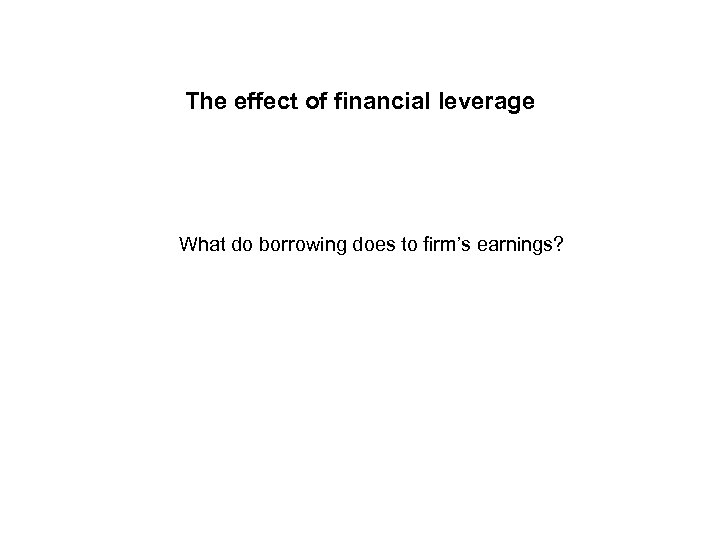The effect of financial leverage What do borrowing does to firm’s earnings?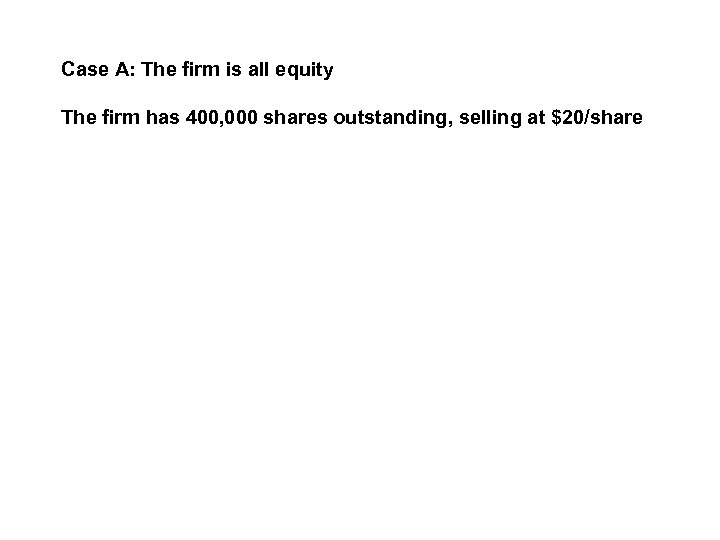Case A: The firm is all equity The firm has 400, 000 shares outstanding, selling at \$20/share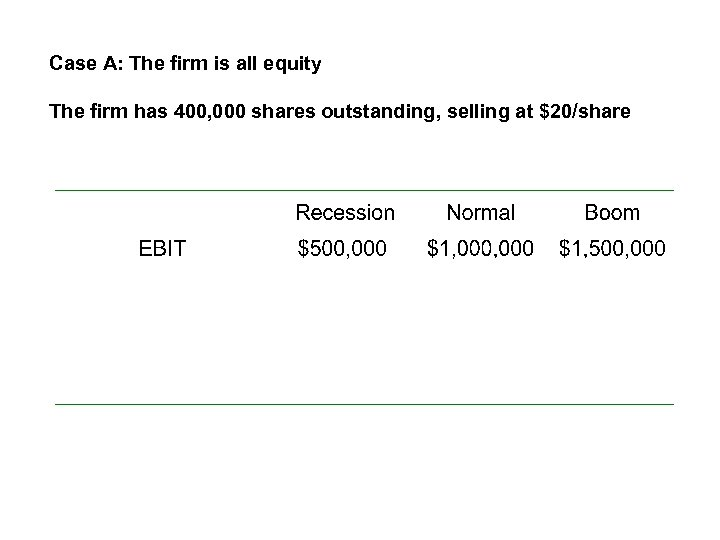Case A: The firm is all equity The firm has 400, 000 shares outstanding, selling at \$20/share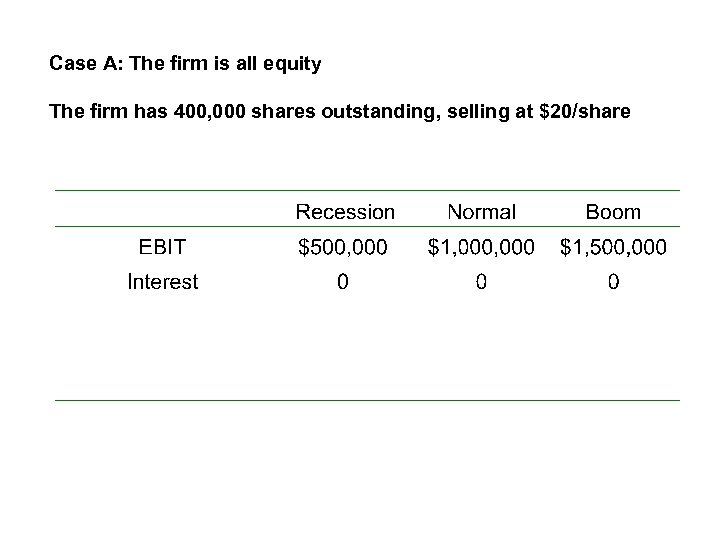Case A: The firm is all equity The firm has 400, 000 shares outstanding, selling at \$20/share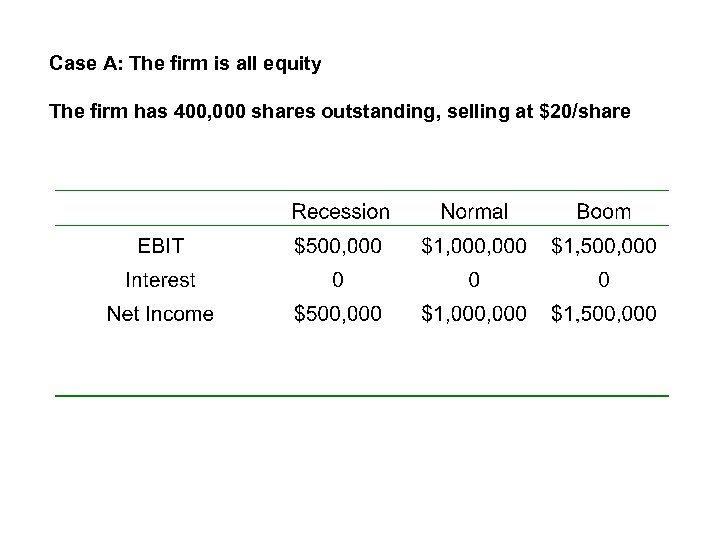Case A: The firm is all equity The firm has 400, 000 shares outstanding, selling at \$20/share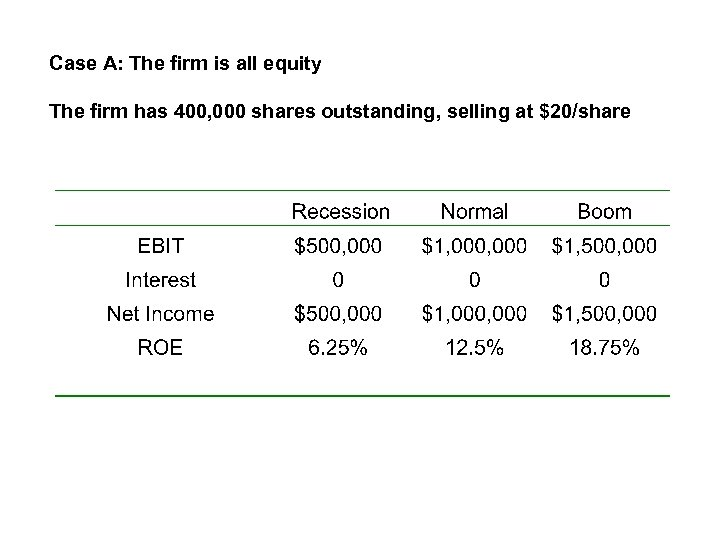Case A: The firm is all equity The firm has 400, 000 shares outstanding, selling at \$20/share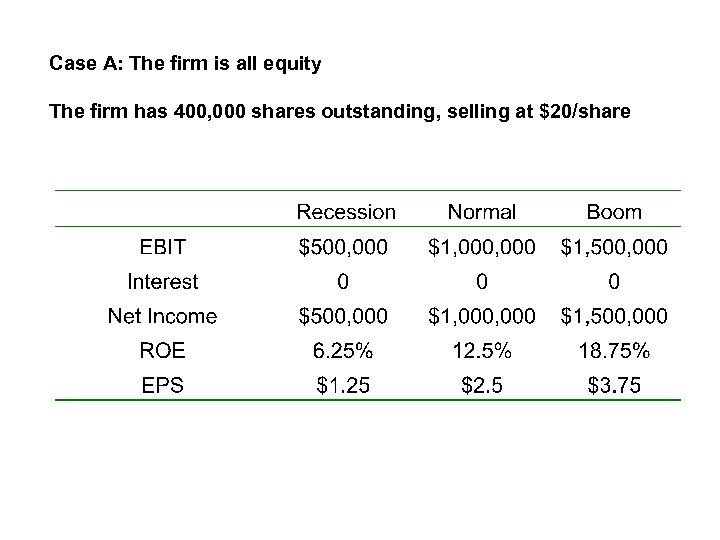Case A: The firm is all equity The firm has 400, 000 shares outstanding, selling at \$20/share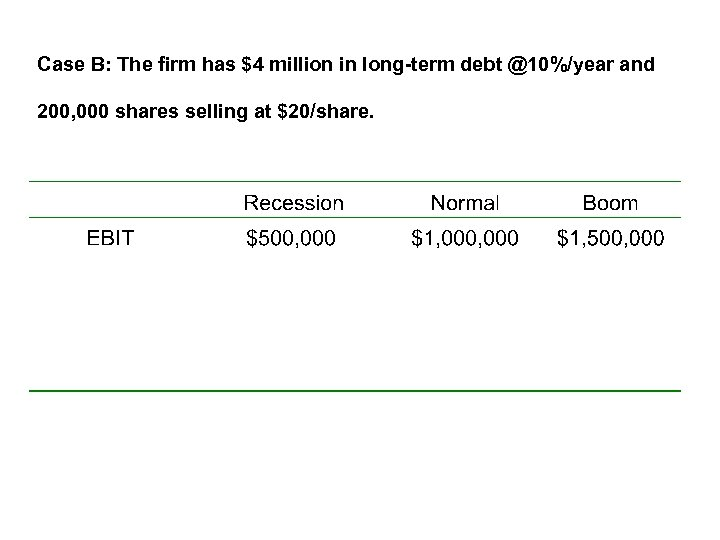Case B: The firm has \$4 million in long-term debt @10%/year and 200, 000 shares selling at \$20/share.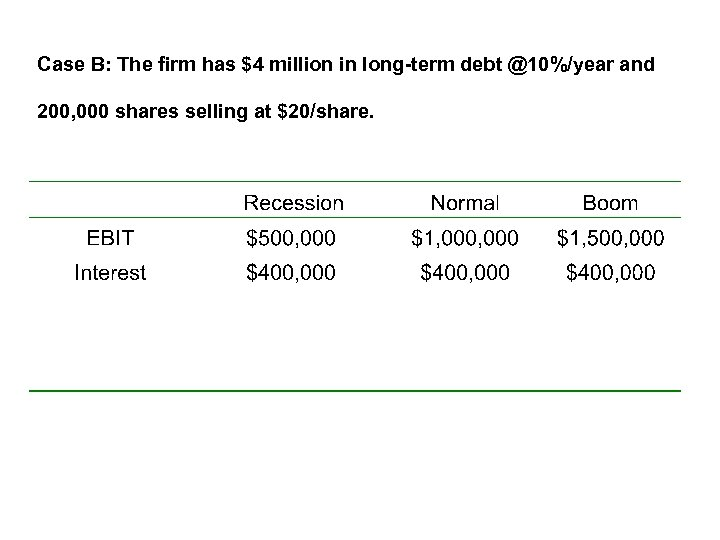Case B: The firm has \$4 million in long-term debt @10%/year and 200, 000 shares selling at \$20/share.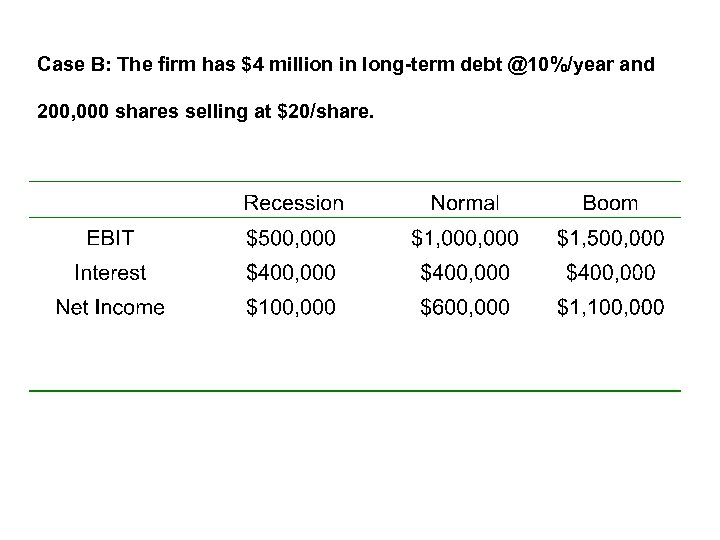Case B: The firm has \$4 million in long-term debt @10%/year and 200, 000 shares selling at \$20/share.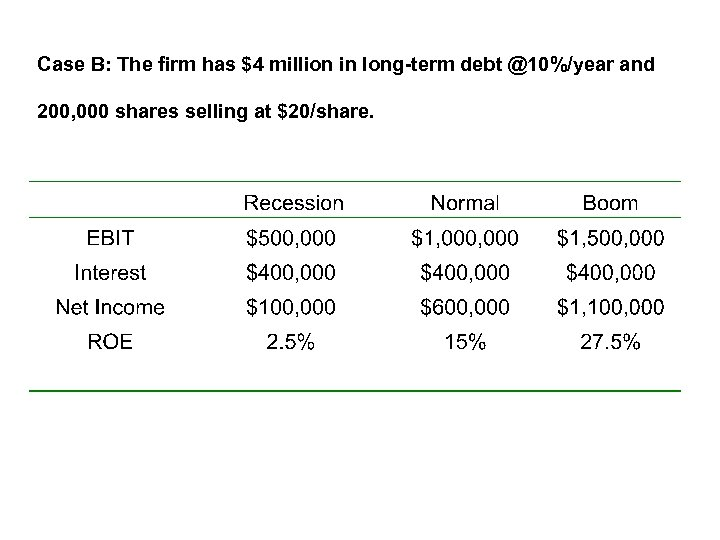Case B: The firm has \$4 million in long-term debt @10%/year and 200, 000 shares selling at \$20/share.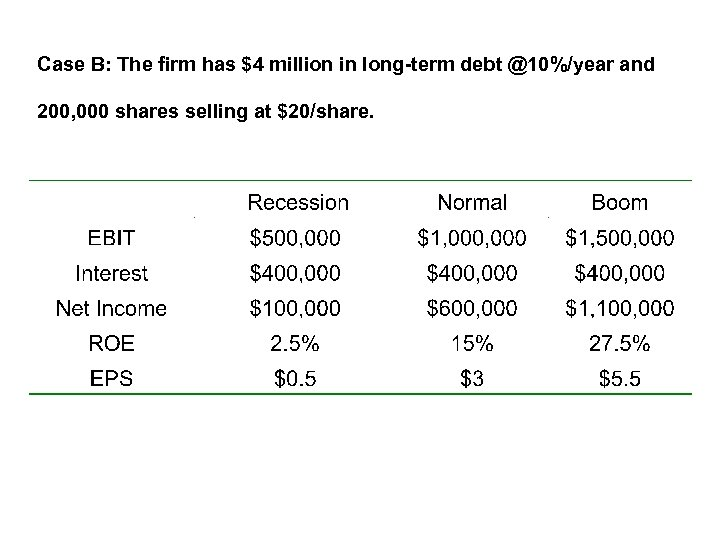Case B: The firm has \$4 million in long-term debt @10%/year and 200, 000 shares selling at \$20/share.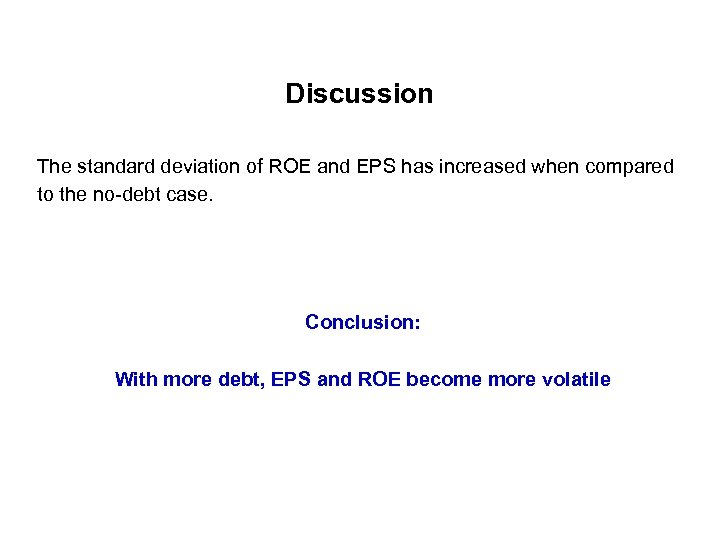Discussion The standard deviation of ROE and EPS has increased when compared to the no-debt case. Conclusion: With more debt, EPS and ROE become more volatile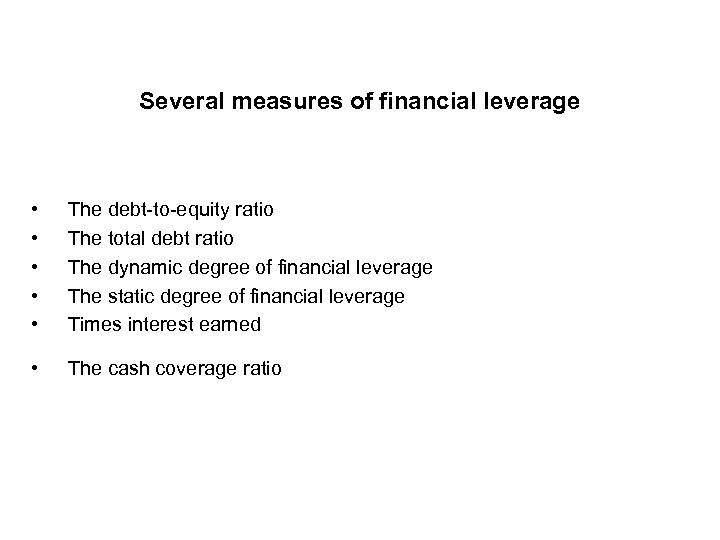Several measures of financial leverage • • • The debt-to-equity ratio The total debt ratio The dynamic degree of financial leverage The static degree of financial leverage Times interest earned • The cash coverage ratio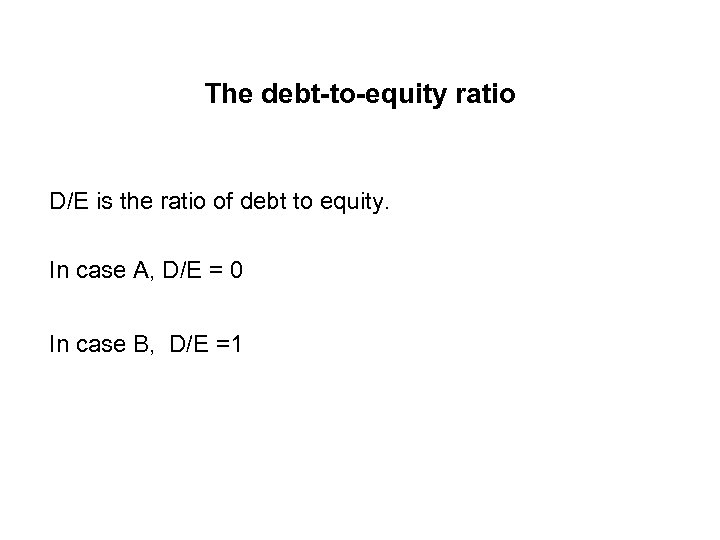The debt-to-equity ratio D/E is the ratio of debt to equity. In case A, D/E = 0 In case B, D/E =1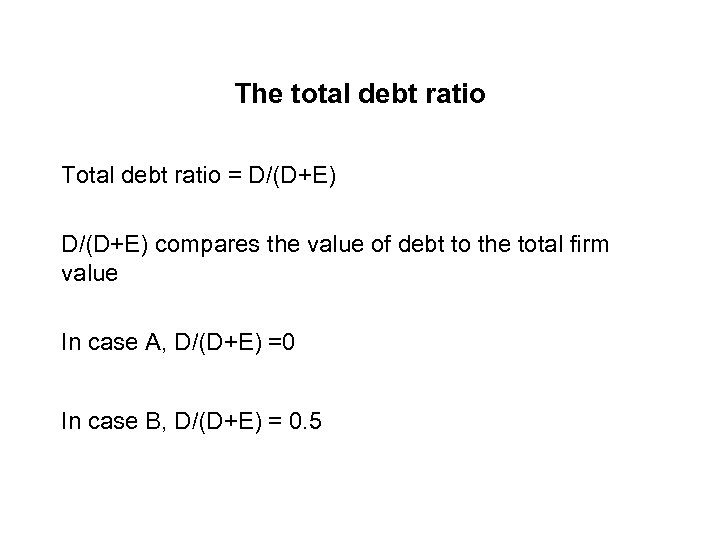The total debt ratio Total debt ratio = D/(D+E) compares the value of debt to the total firm value In case A, D/(D+E) =0 In case B, D/(D+E) = 0. 5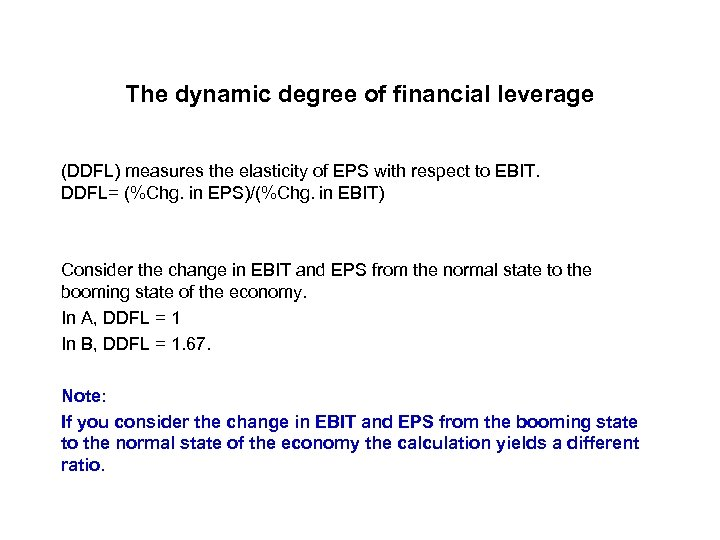The dynamic degree of financial leverage (DDFL) measures the elasticity of EPS with respect to EBIT. DDFL= (%Chg. in EPS)/(%Chg. in EBIT) Consider the change in EBIT and EPS from the normal state to the booming state of the economy. In A, DDFL = 1 In B, DDFL = 1. 67. Note: If you consider the change in EBIT and EPS from the booming state to the normal state of the economy the calculation yields a different ratio.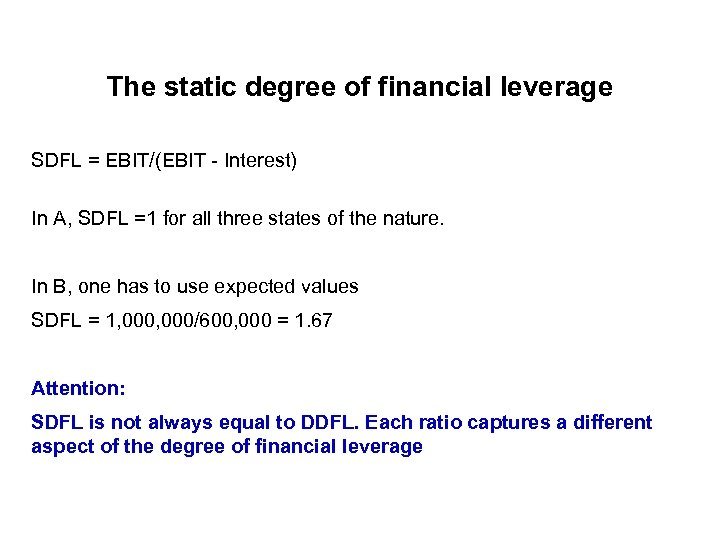The static degree of financial leverage SDFL = EBIT/(EBIT - Interest) In A, SDFL =1 for all three states of the nature. In B, one has to use expected values SDFL = 1, 000/600, 000 = 1. 67 Attention: SDFL is not always equal to DDFL. Each ratio captures a different aspect of the degree of financial leverage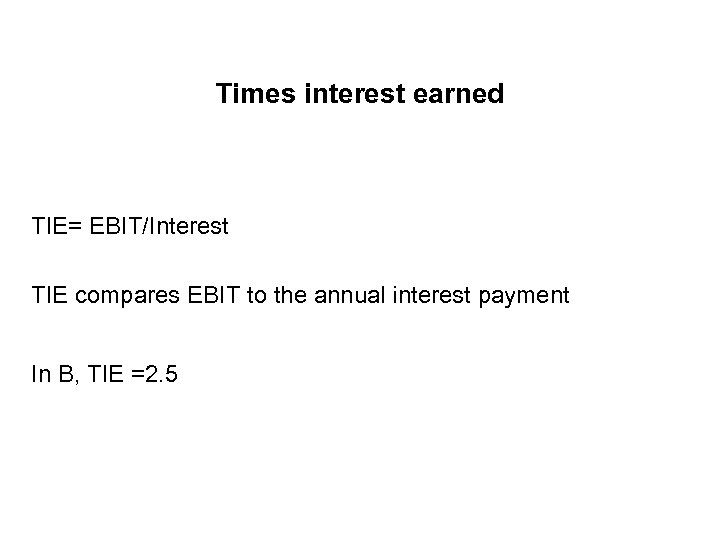Times interest earned TIE= EBIT/Interest TIE compares EBIT to the annual interest payment In B, TIE =2. 5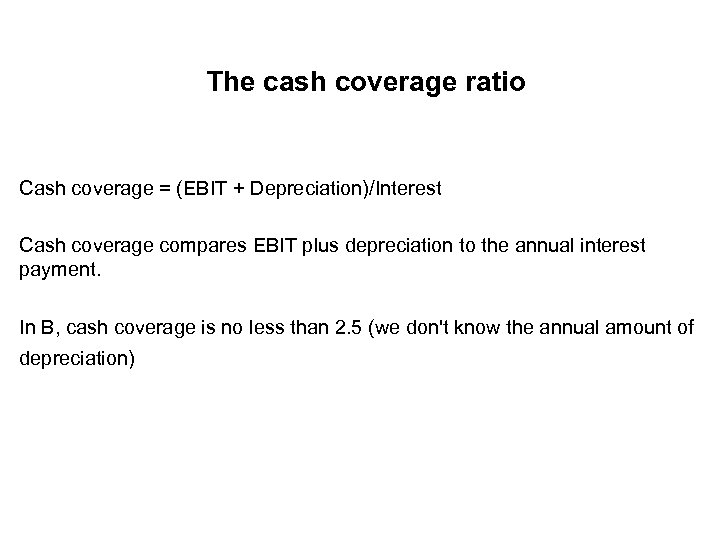The cash coverage ratio Cash coverage = (EBIT + Depreciation)/Interest Cash coverage compares EBIT plus depreciation to the annual interest payment. In B, cash coverage is no less than 2. 5 (we don't know the annual amount of depreciation)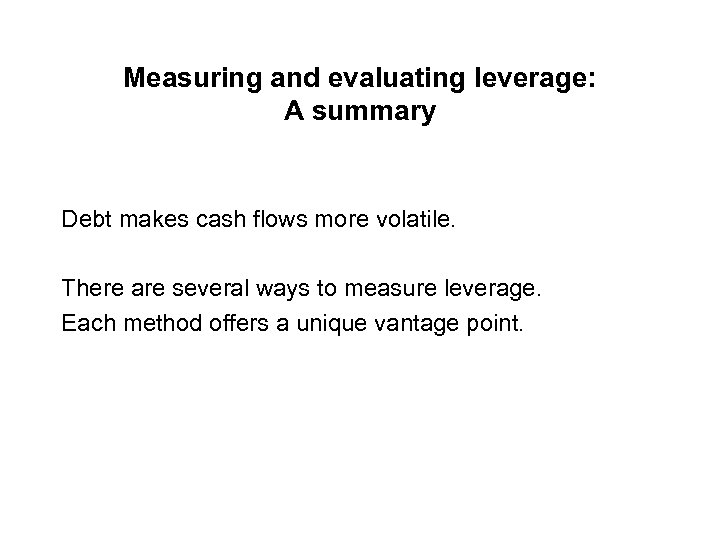Measuring and evaluating leverage: A summary Debt makes cash flows more volatile. There are several ways to measure leverage. Each method offers a unique vantage point.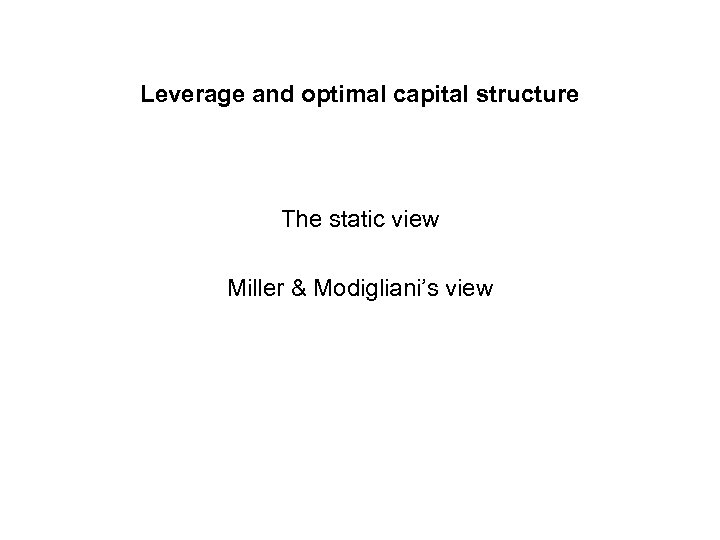Leverage and optimal capital structure The static view Miller & Modigliani’s view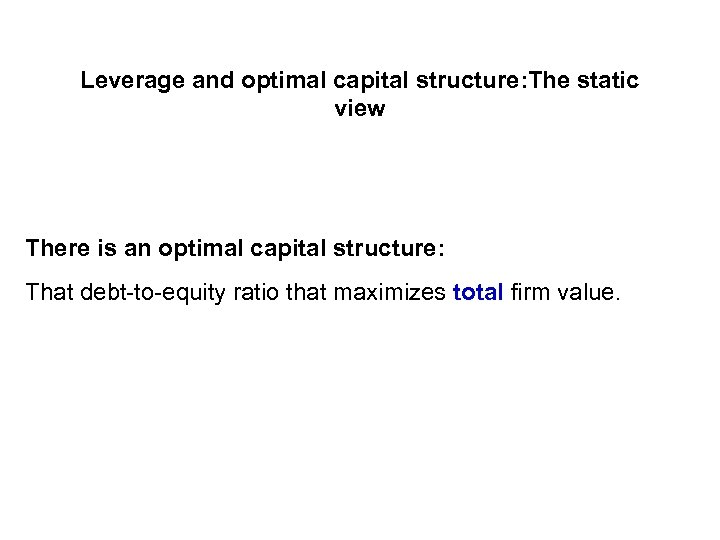Leverage and optimal capital structure: The static view There is an optimal capital structure: That debt-to-equity ratio that maximizes total firm value.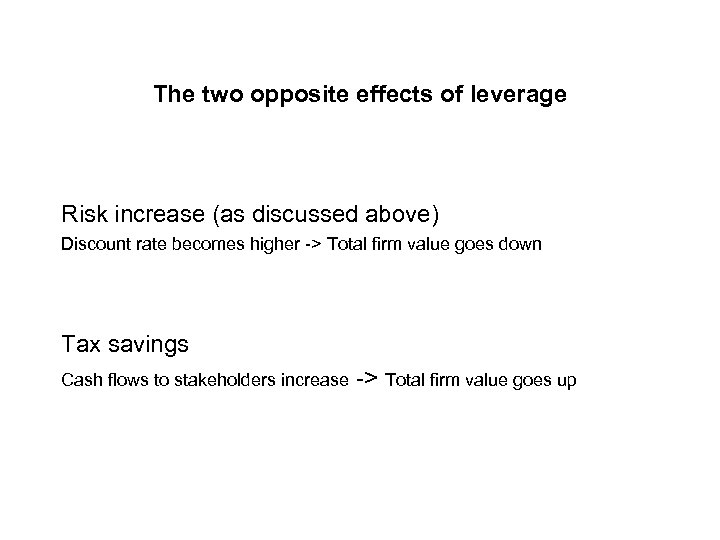The two opposite effects of leverage Risk increase (as discussed above) Discount rate becomes higher -> Total firm value goes down Tax savings Cash flows to stakeholders increase -> Total firm value goes up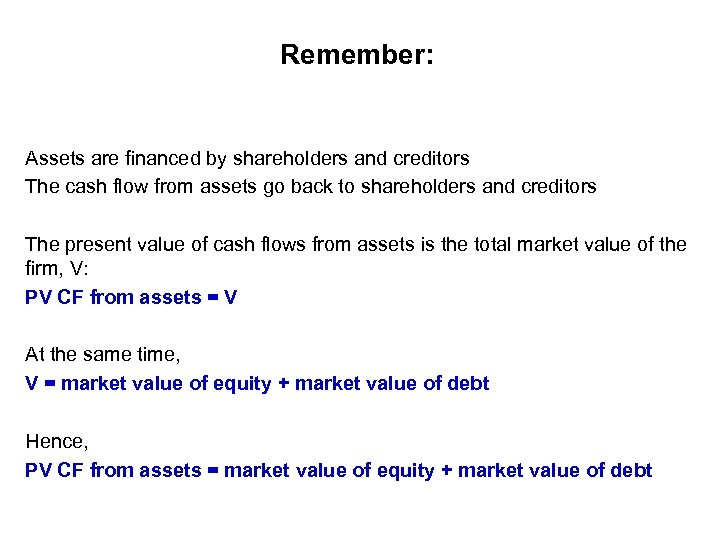Remember: Assets are financed by shareholders and creditors The cash flow from assets go back to shareholders and creditors The present value of cash flows from assets is the total market value of the firm, V: PV CF from assets = V At the same time, V = market value of equity + market value of debt Hence, PV CF from assets = market value of equity + market value of debt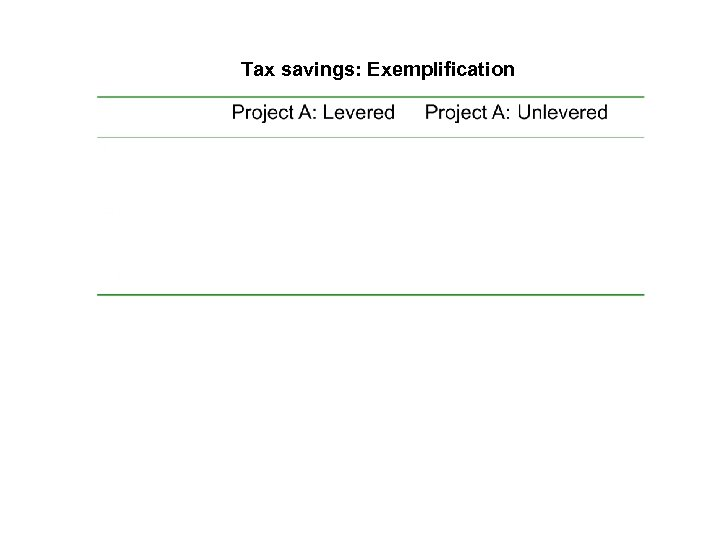Tax savings: Exemplification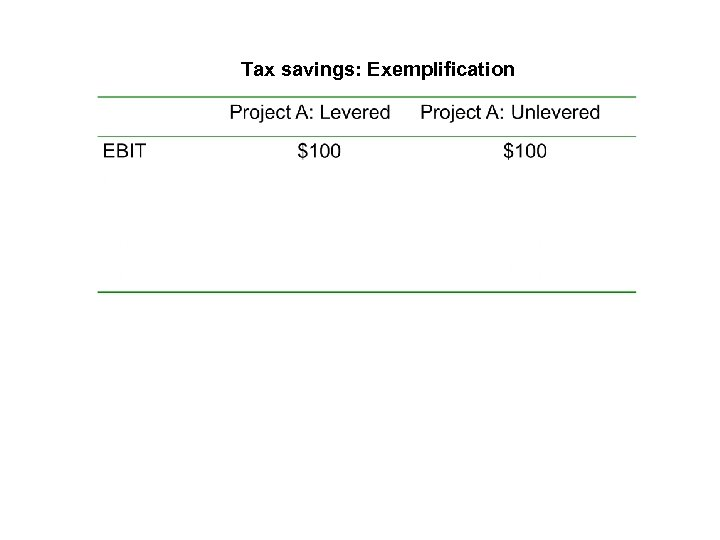Tax savings: Exemplification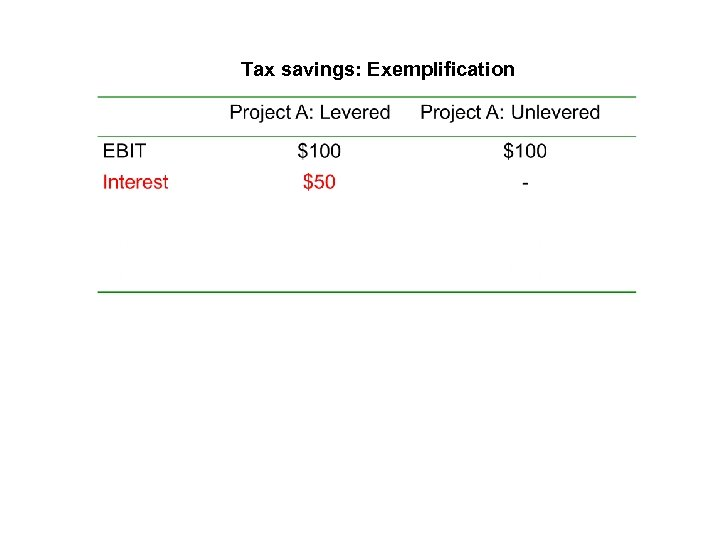Tax savings: Exemplification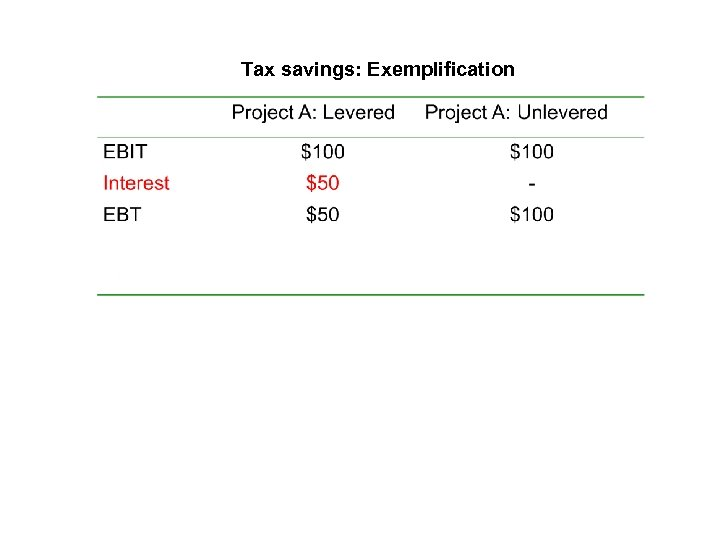Tax savings: Exemplification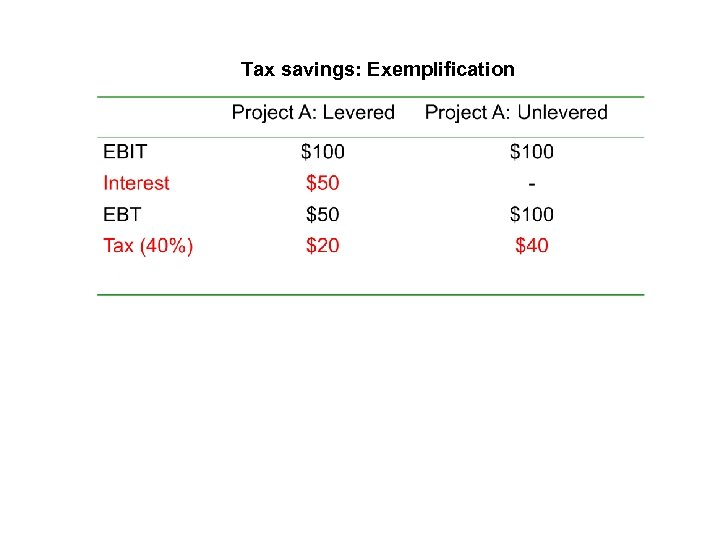Tax savings: Exemplification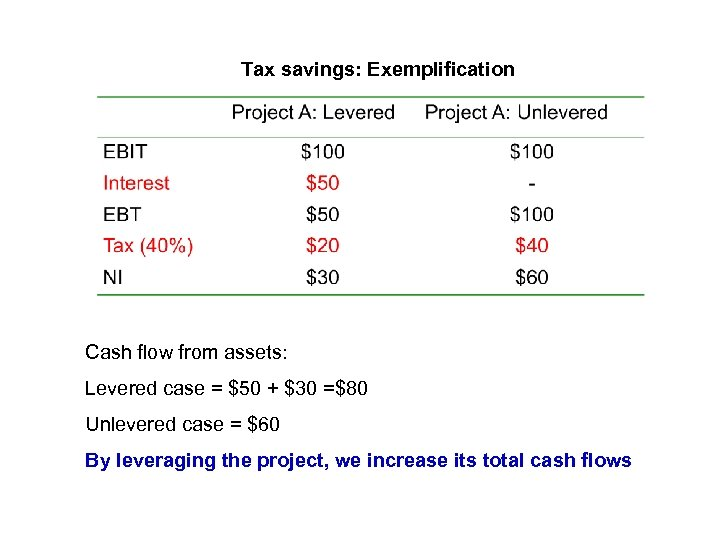Tax savings: Exemplification Cash flow from assets: Levered case = \$50 + \$30 =\$80 Unlevered case = \$60 By leveraging the project, we increase its total cash flows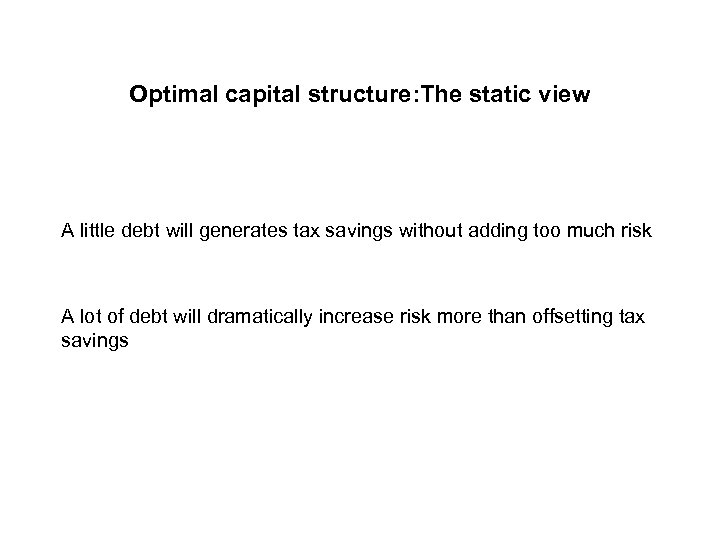Optimal capital structure: The static view A little debt will generates tax savings without adding too much risk A lot of debt will dramatically increase risk more than offsetting tax savings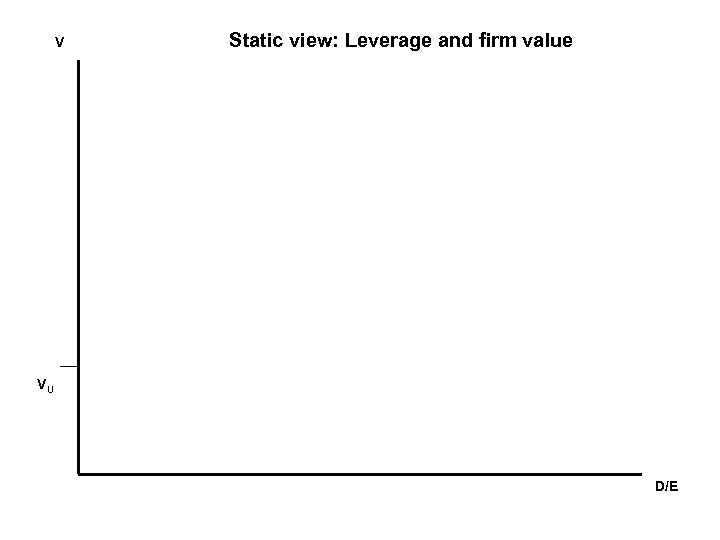V Static view: Leverage and firm value VU D/E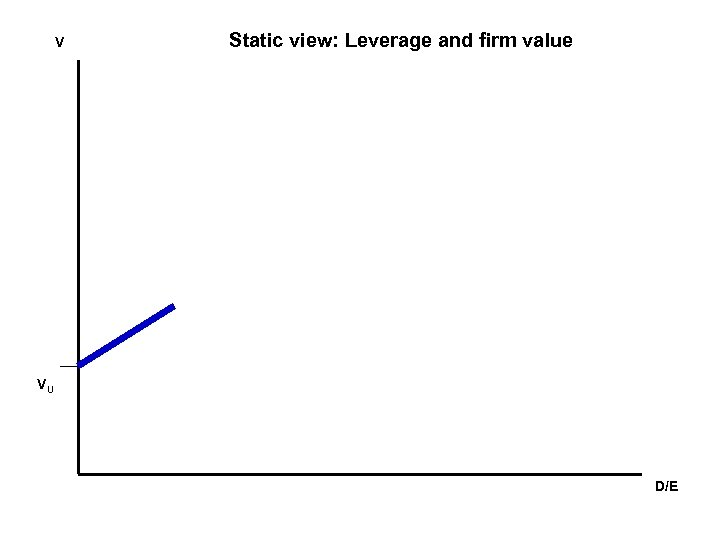V Static view: Leverage and firm value VU D/E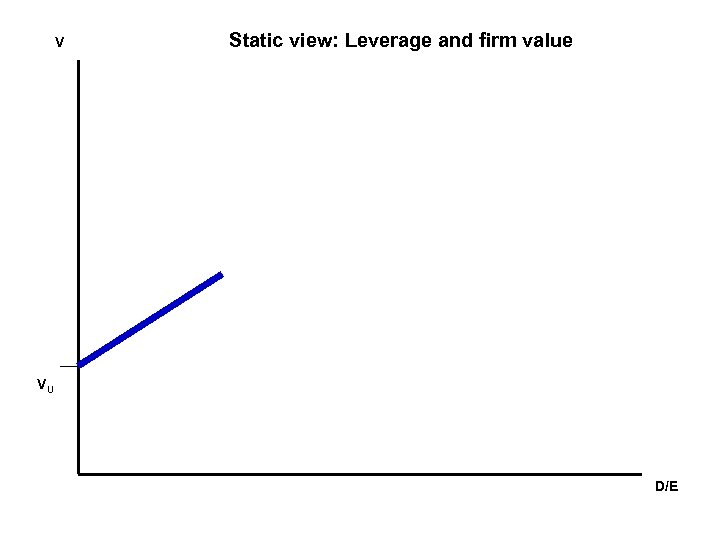V Static view: Leverage and firm value VU D/E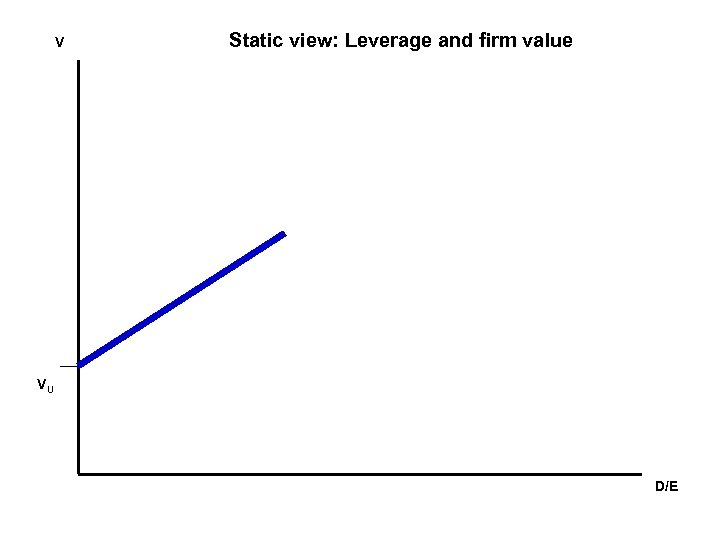V Static view: Leverage and firm value VU D/E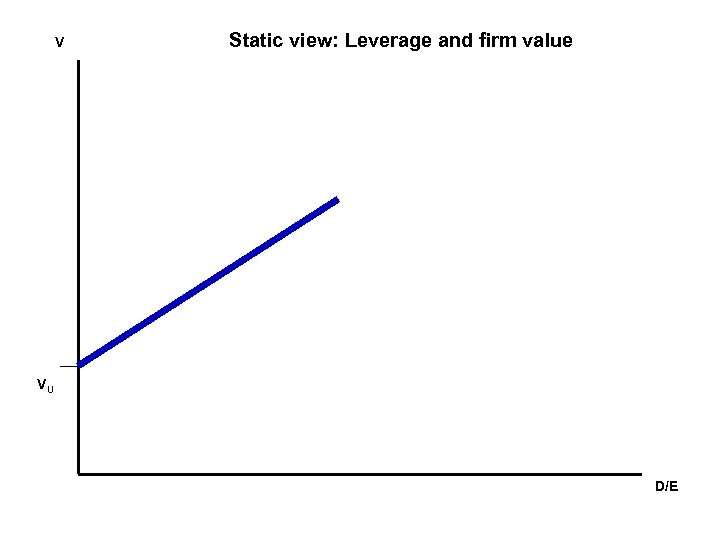V Static view: Leverage and firm value VU D/E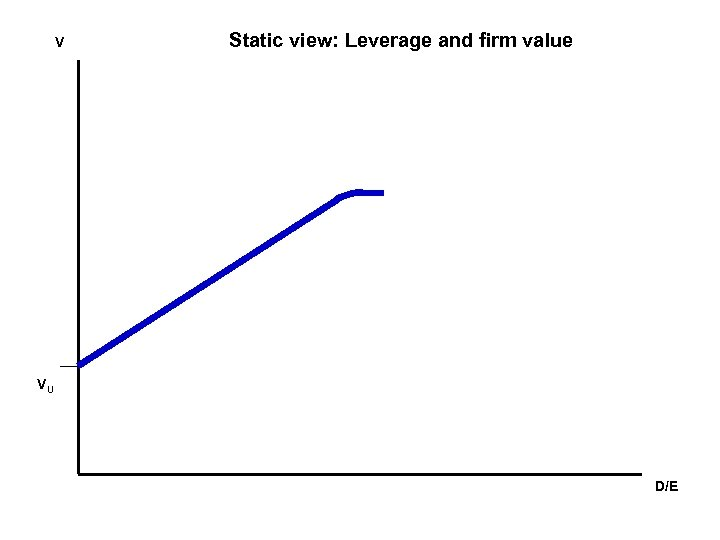V Static view: Leverage and firm value VU D/E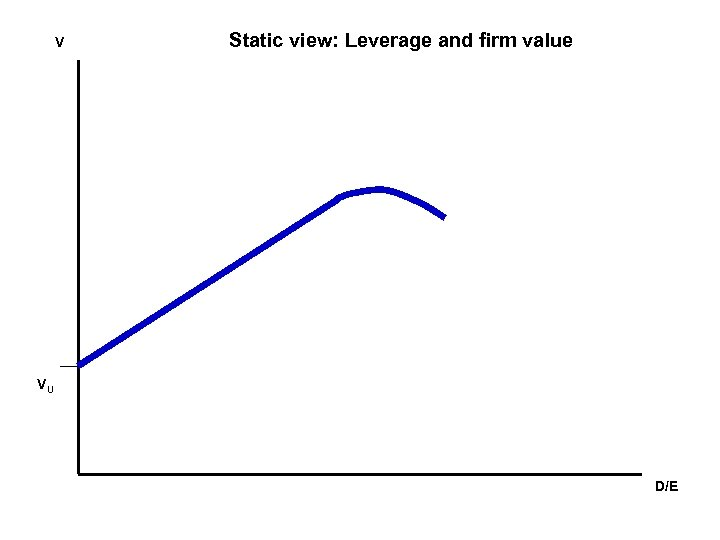V Static view: Leverage and firm value VU D/E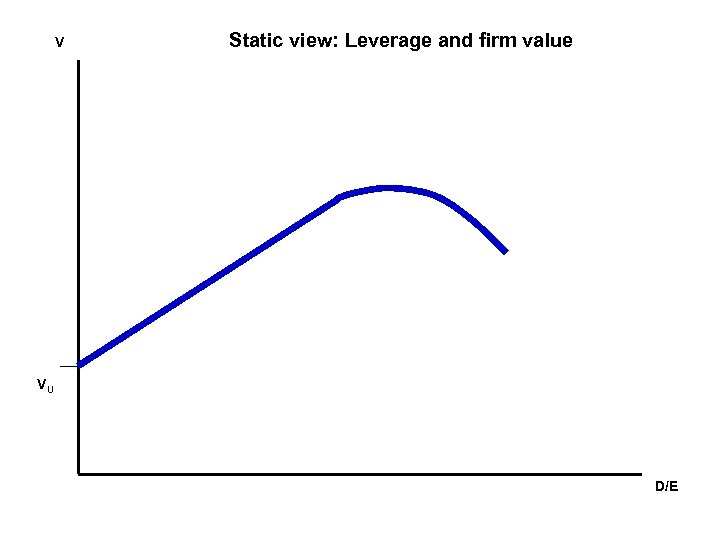V Static view: Leverage and firm value VU D/E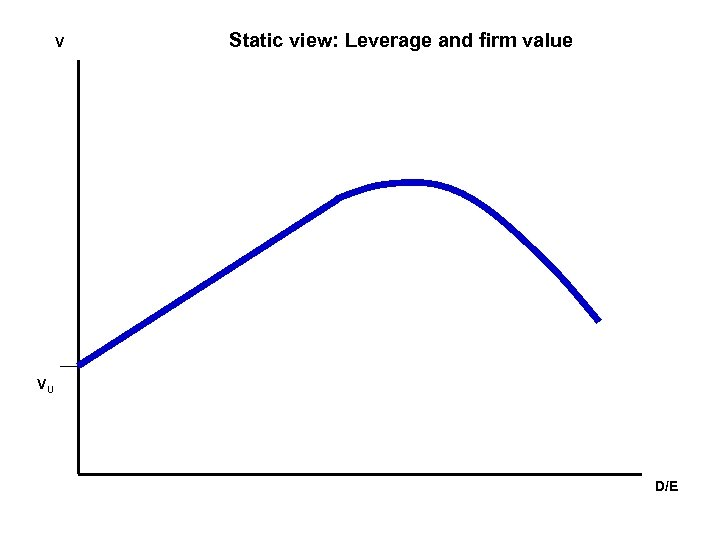V Static view: Leverage and firm value VU D/E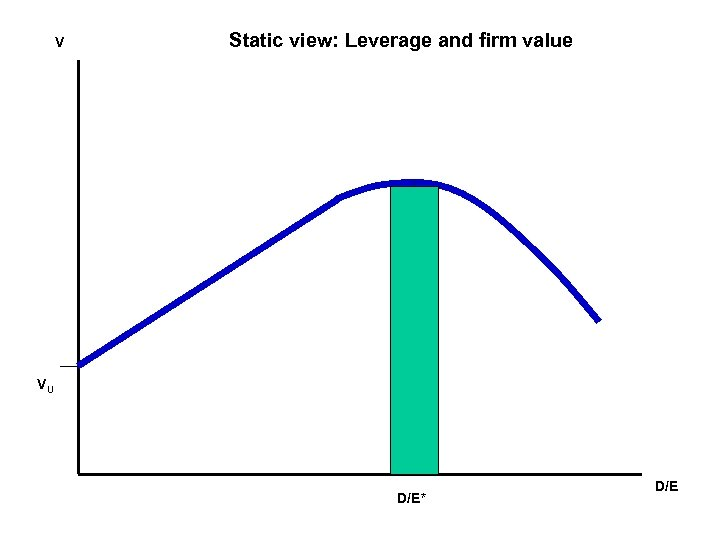V Static view: Leverage and firm value VU D/E* D/E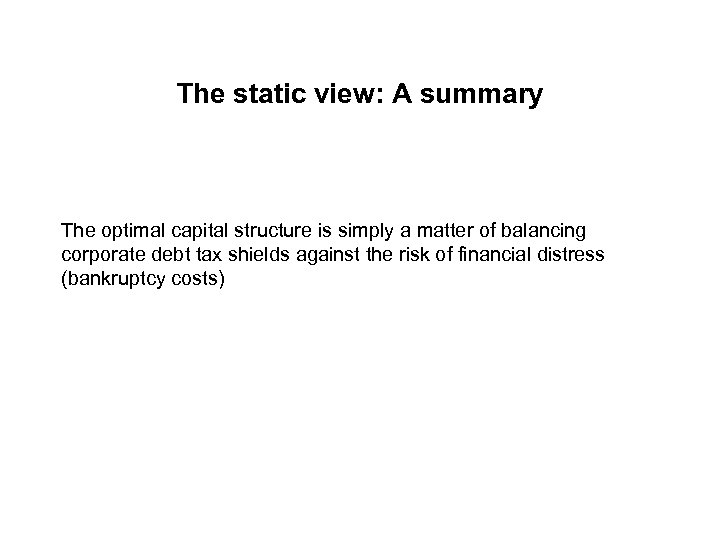The static view: A summary The optimal capital structure is simply a matter of balancing corporate debt tax shields against the risk of financial distress (bankruptcy costs)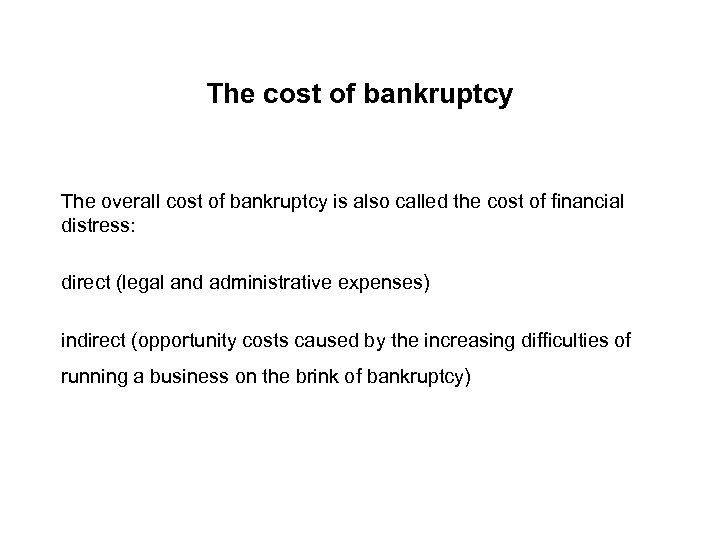The cost of bankruptcy The overall cost of bankruptcy is also called the cost of financial distress: direct (legal and administrative expenses) indirect (opportunity costs caused by the increasing difficulties of running a business on the brink of bankruptcy)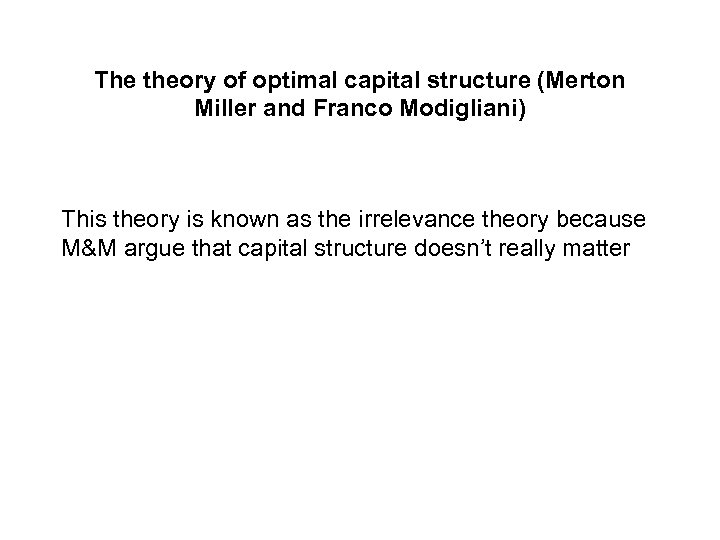The theory of optimal capital structure (Merton Miller and Franco Modigliani) This theory is known as the irrelevance theory because M&M argue that capital structure doesn’t really matter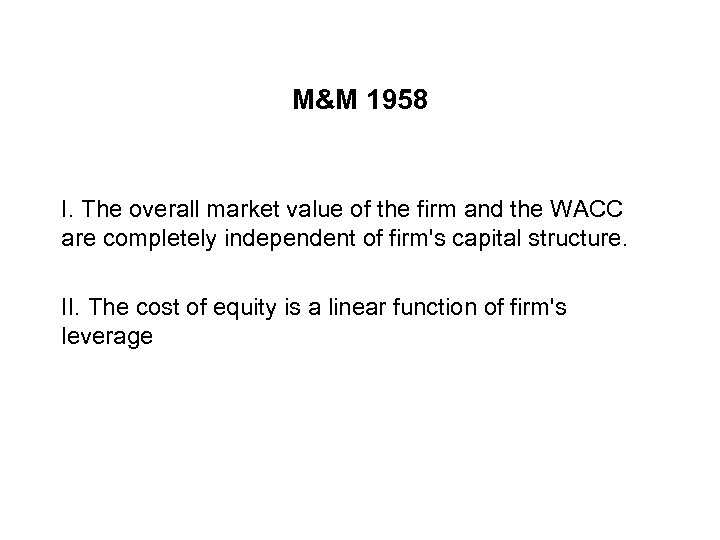M&M 1958 I. The overall market value of the firm and the WACC are completely independent of firm's capital structure. II. The cost of equity is a linear function of firm's leverage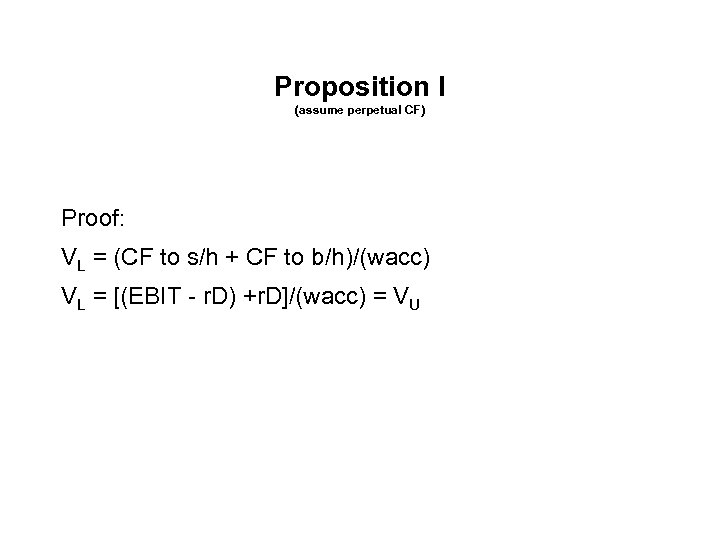Proposition I (assume perpetual CF) Proof: VL = (CF to s/h + CF to b/h)/(wacc) VL = [(EBIT - r. D) +r. D]/(wacc) = VU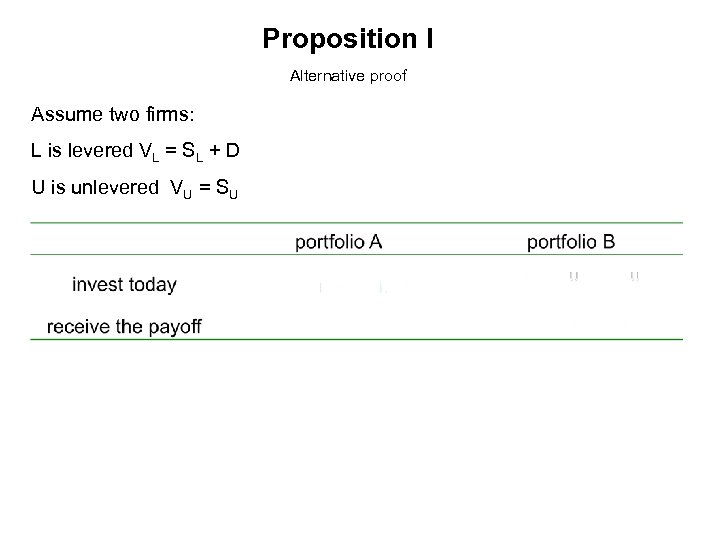Proposition I Alternative proof Assume two firms: L is levered VL = SL + D U is unlevered VU = SU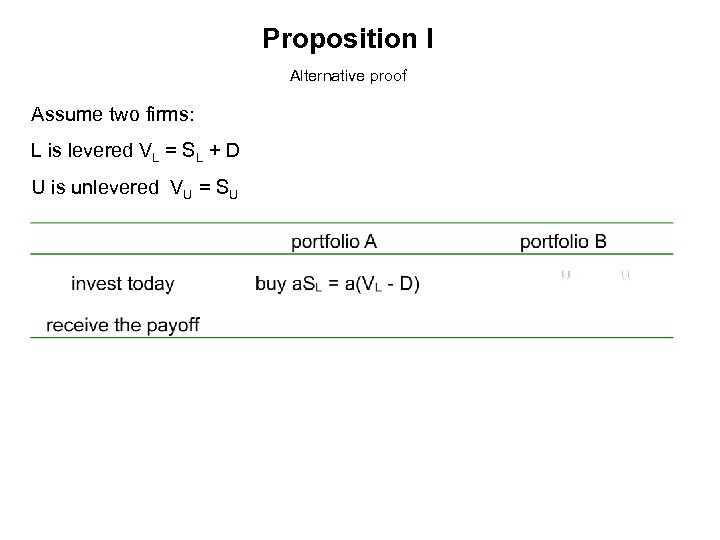Proposition I Alternative proof Assume two firms: L is levered VL = SL + D U is unlevered VU = SU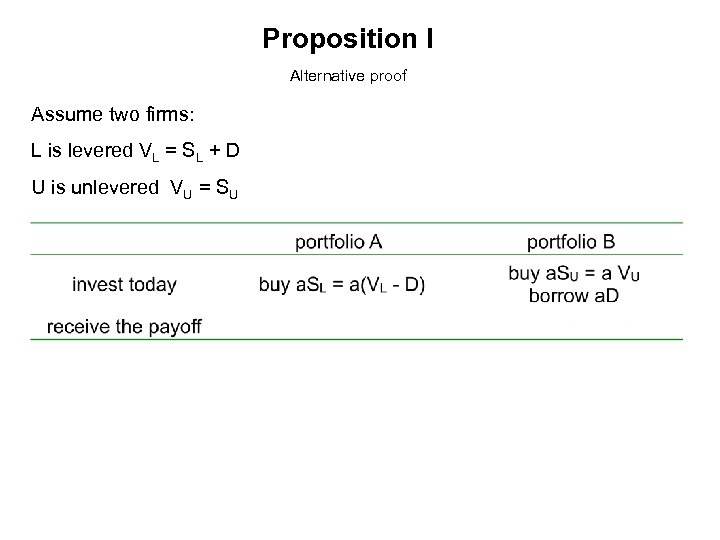Proposition I Alternative proof Assume two firms: L is levered VL = SL + D U is unlevered VU = SU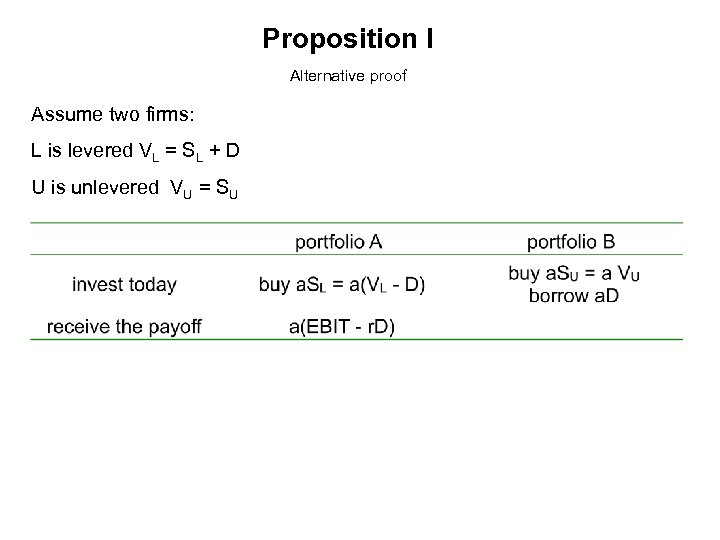Proposition I Alternative proof Assume two firms: L is levered VL = SL + D U is unlevered VU = SU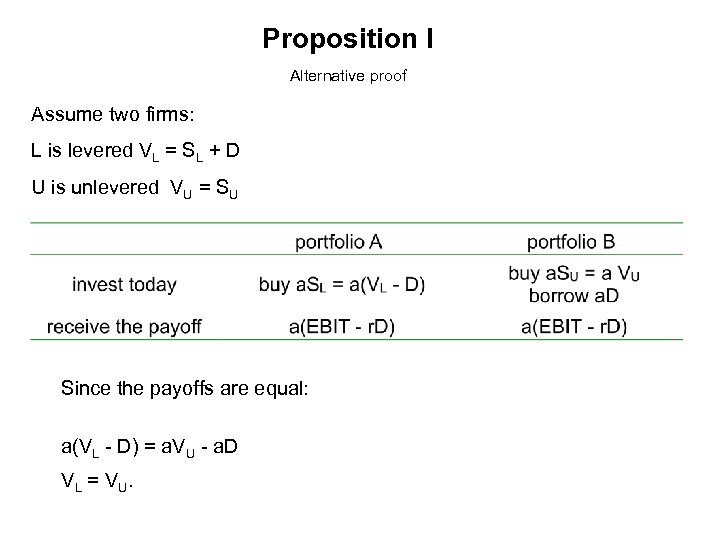Proposition I Alternative proof Assume two firms: L is levered VL = SL + D U is unlevered VU = SU Since the payoffs are equal: a(VL - D) = a. VU - a. D VL = VU.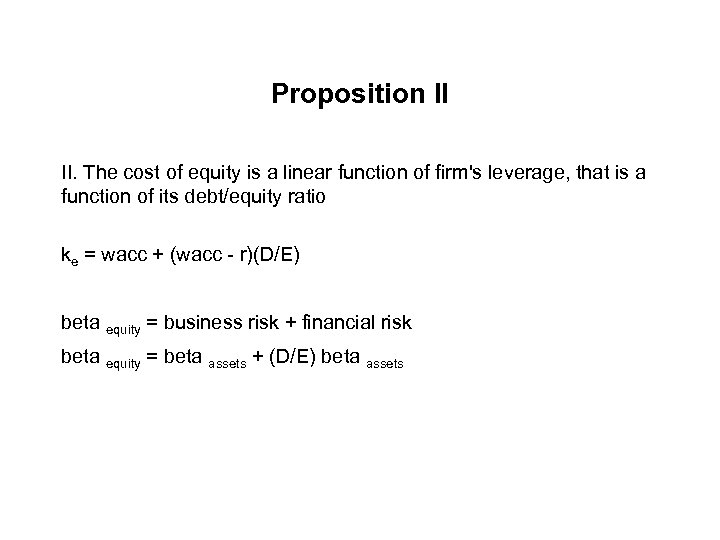Proposition II II. The cost of equity is a linear function of firm's leverage, that is a function of its debt/equity ratio ke = wacc + (wacc - r)(D/E) beta equity = business risk + financial risk beta equity = beta assets + (D/E) beta assets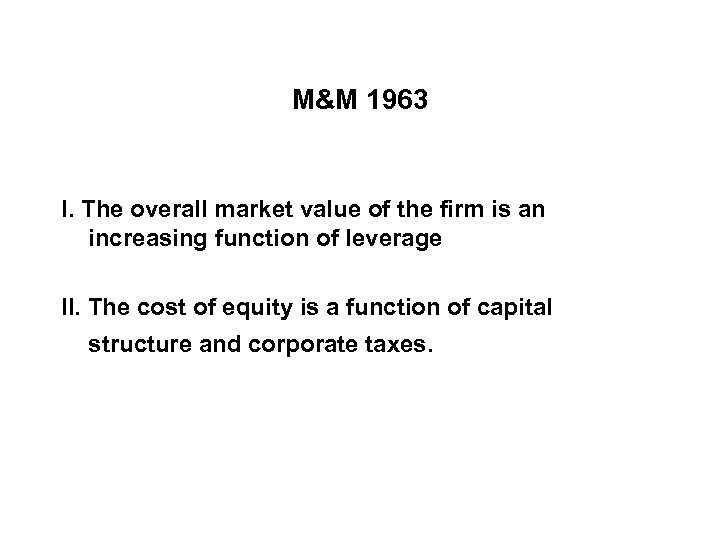M&M 1963 I. The overall market value of the firm is an increasing function of leverage II. The cost of equity is a function of capital structure and corporate taxes.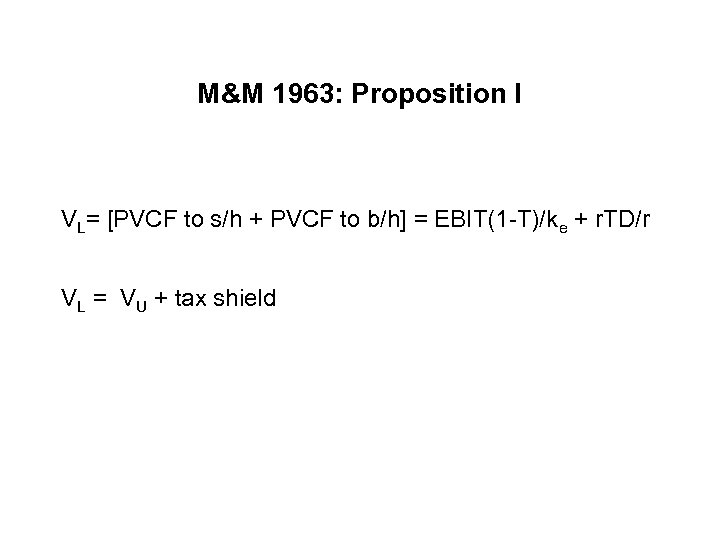M&M 1963: Proposition I VL= [PVCF to s/h + PVCF to b/h] = EBIT(1 -T)/ke + r. TD/r VL = VU + tax shield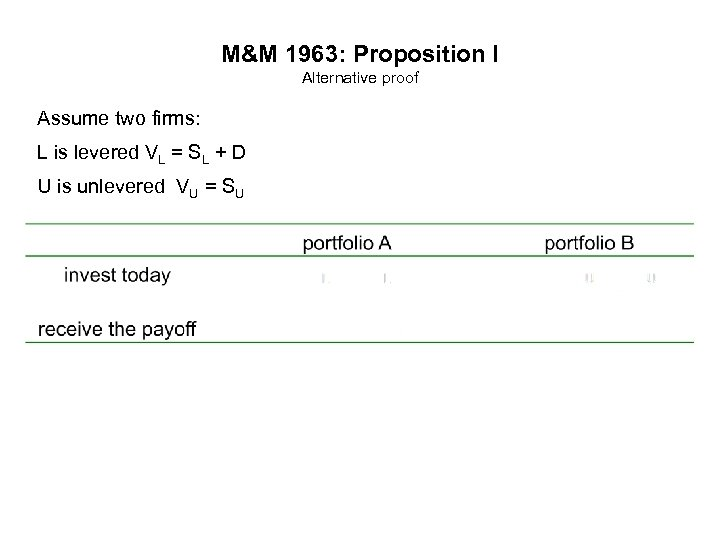M&M 1963: Proposition I Alternative proof Assume two firms: L is levered VL = SL + D U is unlevered VU = SU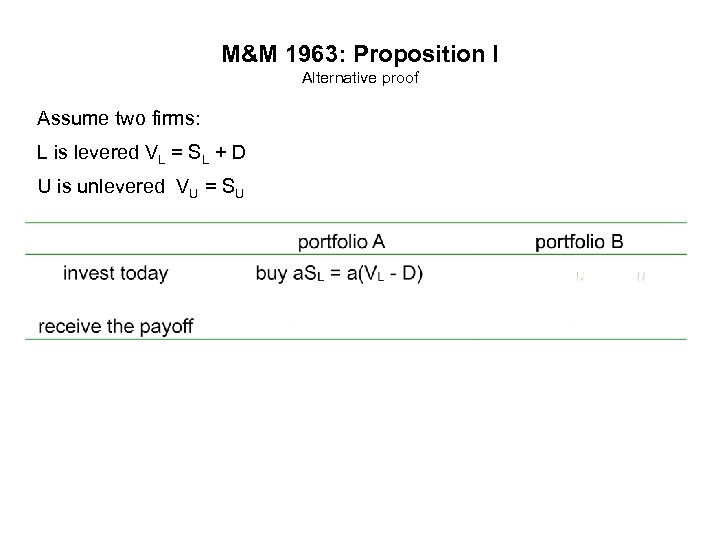M&M 1963: Proposition I Alternative proof Assume two firms: L is levered VL = SL + D U is unlevered VU = SU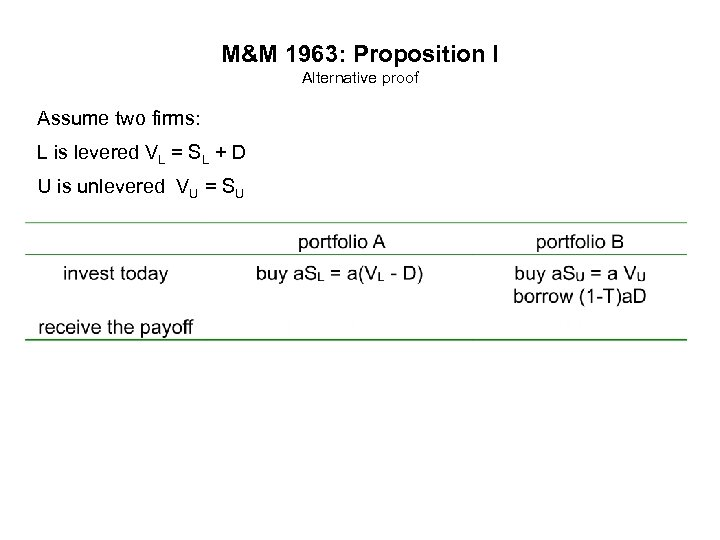M&M 1963: Proposition I Alternative proof Assume two firms: L is levered VL = SL + D U is unlevered VU = SU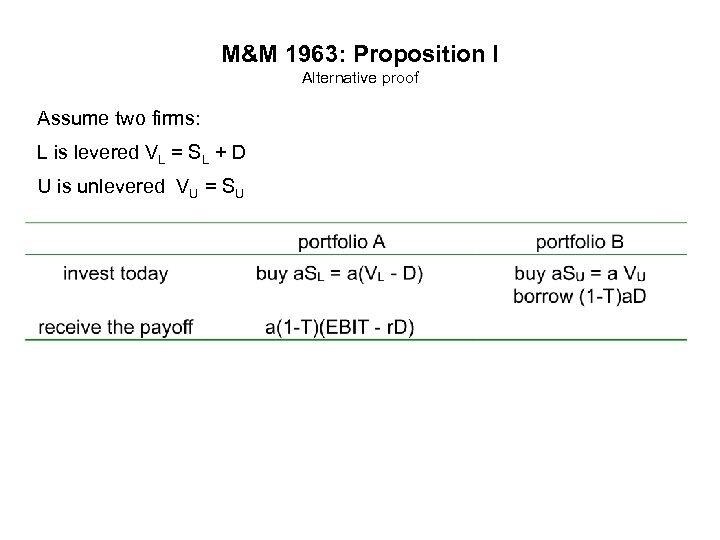M&M 1963: Proposition I Alternative proof Assume two firms: L is levered VL = SL + D U is unlevered VU = SU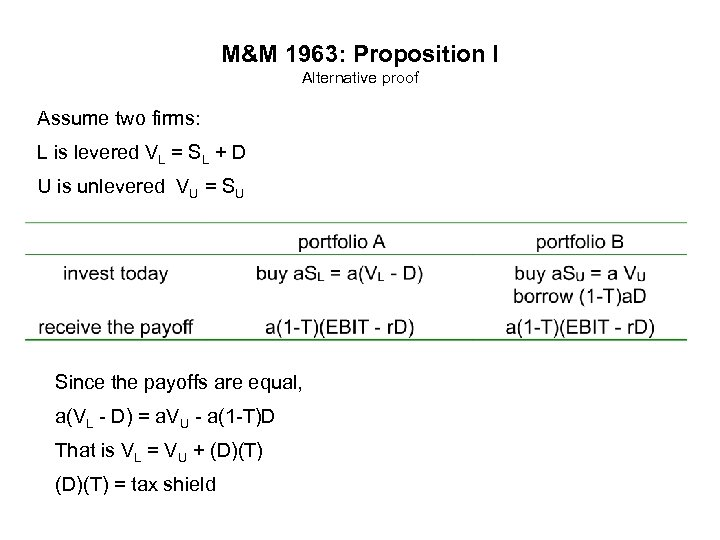M&M 1963: Proposition I Alternative proof Assume two firms: L is levered VL = SL + D U is unlevered VU = SU Since the payoffs are equal, a(VL - D) = a. VU - a(1 -T)D That is VL = VU + (D)(T) = tax shield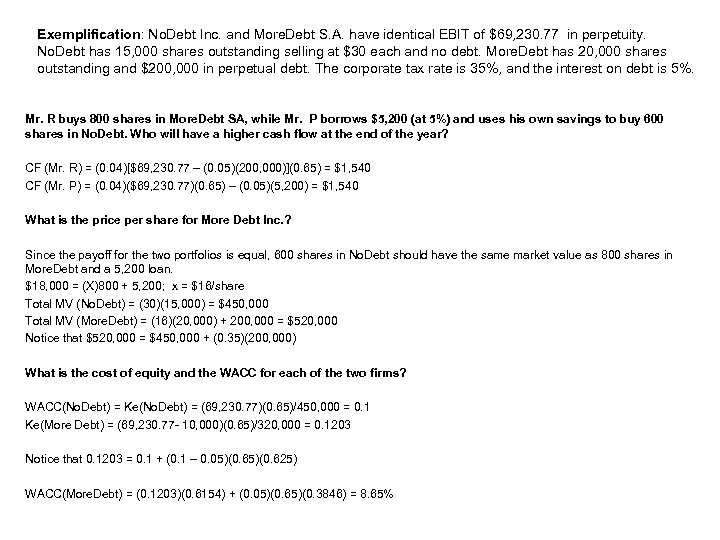Exemplification: No. Debt Inc. and More. Debt S. A. have identical EBIT of \$69, 230. 77 in perpetuity. No. Debt has 15, 000 shares outstanding selling at \$30 each and no debt. More. Debt has 20, 000 shares outstanding and \$200, 000 in perpetual debt. The corporate tax rate is 35%, and the interest on debt is 5%. Mr. R buys 800 shares in More. Debt SA, while Mr. P borrows \$5, 200 (at 5%) and uses his own savings to buy 600 shares in No. Debt. Who will have a higher cash flow at the end of the year? CF (Mr. R) = (0. 04)[\$69, 230. 77 – (0. 05)(200, 000)](0. 65) = \$1, 540 CF (Mr. P) = (0. 04)(\$69, 230. 77)(0. 65) – (0. 05)(5, 200) = \$1, 540 What is the price per share for More Debt Inc. ? Since the payoff for the two portfolios is equal, 600 shares in No. Debt should have the same market value as 800 shares in More. Debt and a 5, 200 loan. \$18, 000 = (X)800 + 5, 200; x = \$16/share Total MV (No. Debt) = (30)(15, 000) = \$450, 000 Total MV (More. Debt) = (16)(20, 000) + 200, 000 = \$520, 000 Notice that \$520, 000 = \$450, 000 + (0. 35)(200, 000) What is the cost of equity and the WACC for each of the two firms? WACC(No. Debt) = Ke(No. Debt) = (69, 230. 77)(0. 65)/450, 000 = 0. 1 Ke(More Debt) = (69, 230. 77 - 10, 000)(0. 65)/320, 000 = 0. 1203 Notice that 0. 1203 = 0. 1 + (0. 1 – 0. 05)(0. 625) WACC(More. Debt) = (0. 1203)(0. 6154) + (0. 05)(0. 65)(0. 3846) = 8. 65%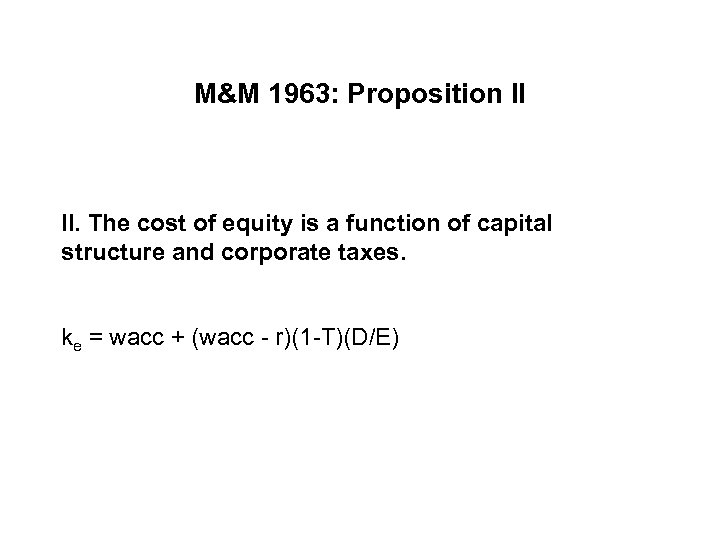M&M 1963: Proposition II II. The cost of equity is a function of capital structure and corporate taxes. ke = wacc + (wacc - r)(1 -T)(D/E)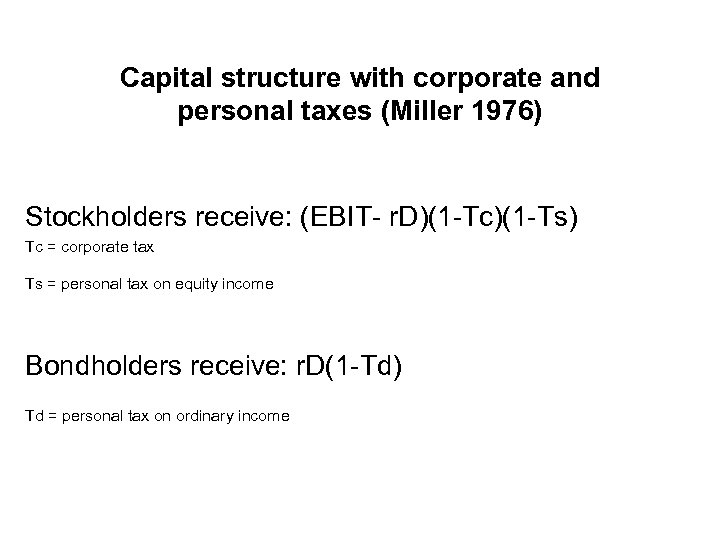Capital structure with corporate and personal taxes (Miller 1976) Stockholders receive: (EBIT- r. D)(1 -Tc)(1 -Ts) Tc = corporate tax Ts = personal tax on equity income Bondholders receive: r. D(1 -Td) Td = personal tax on ordinary income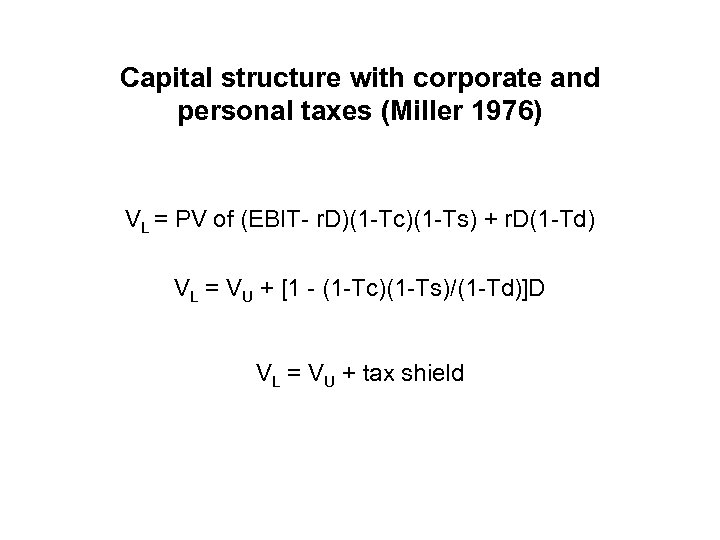Capital structure with corporate and personal taxes (Miller 1976) VL = PV of (EBIT- r. D)(1 -Tc)(1 -Ts) + r. D(1 -Td) VL = VU + [1 - (1 -Tc)(1 -Ts)/(1 -Td)]D VL = VU + tax shield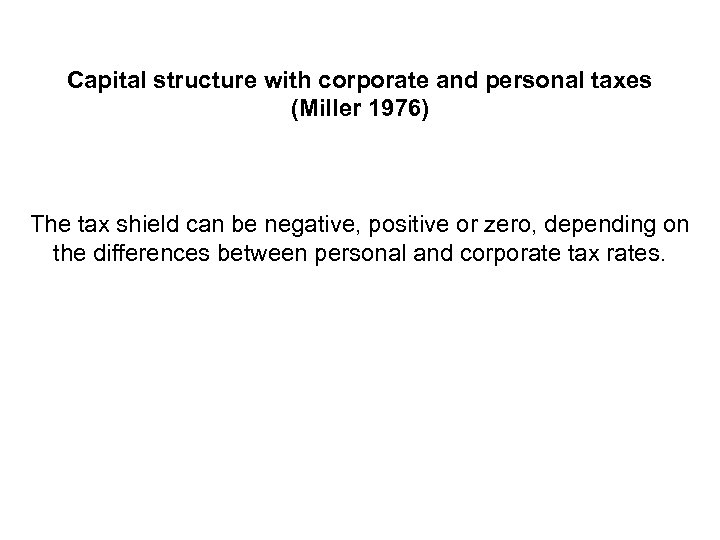Capital structure with corporate and personal taxes (Miller 1976) The tax shield can be negative, positive or zero, depending on the differences between personal and corporate tax rates.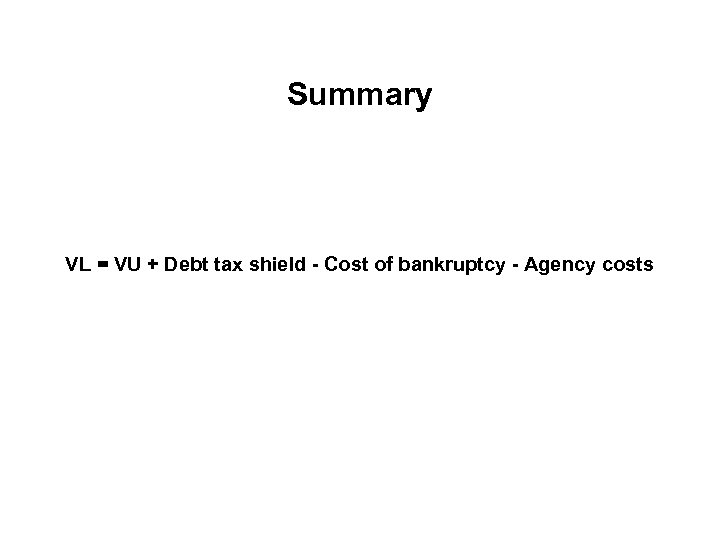Summary VL = VU + Debt tax shield - Cost of bankruptcy - Agency costs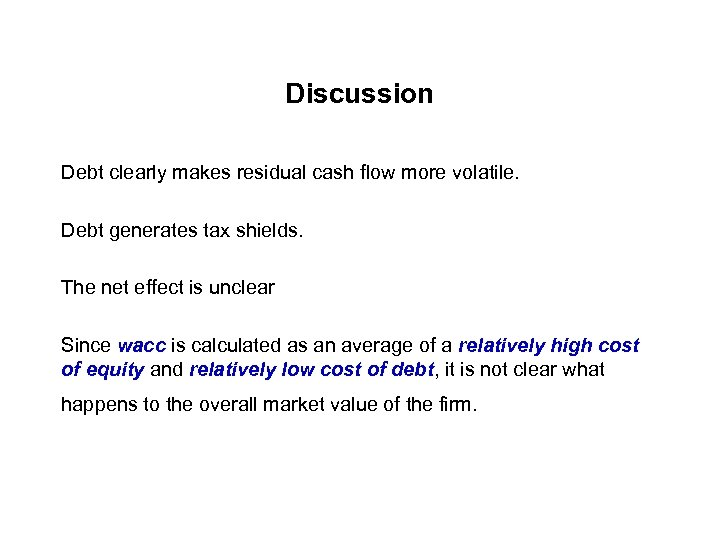Discussion Debt clearly makes residual cash flow more volatile. Debt generates tax shields. The net effect is unclear Since wacc is calculated as an average of a relatively high cost of equity and relatively low cost of debt, it is not clear what happens to the overall market value of the firm.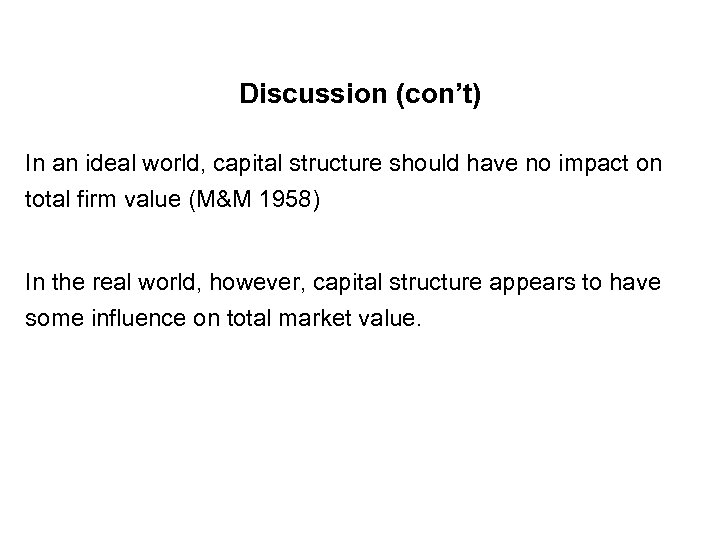Discussion (con’t) In an ideal world, capital structure should have no impact on total firm value (M&M 1958) In the real world, however, capital structure appears to have some influence on total market value.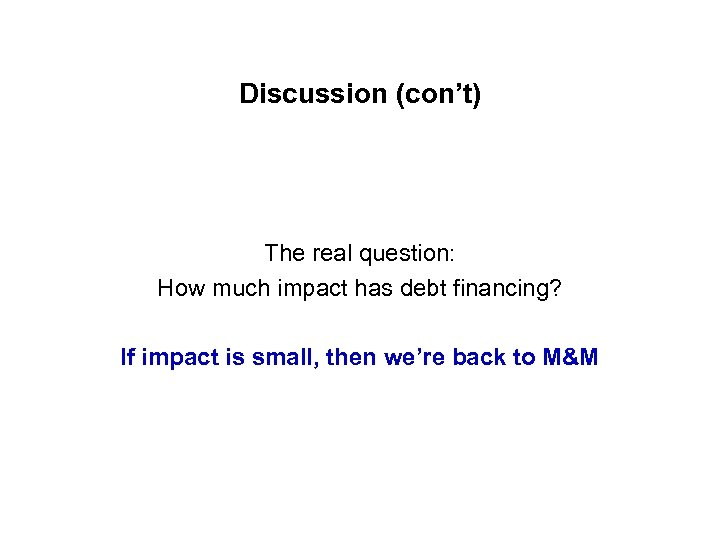Discussion (con’t) The real question: How much impact has debt financing? If impact is small, then we’re back to M&M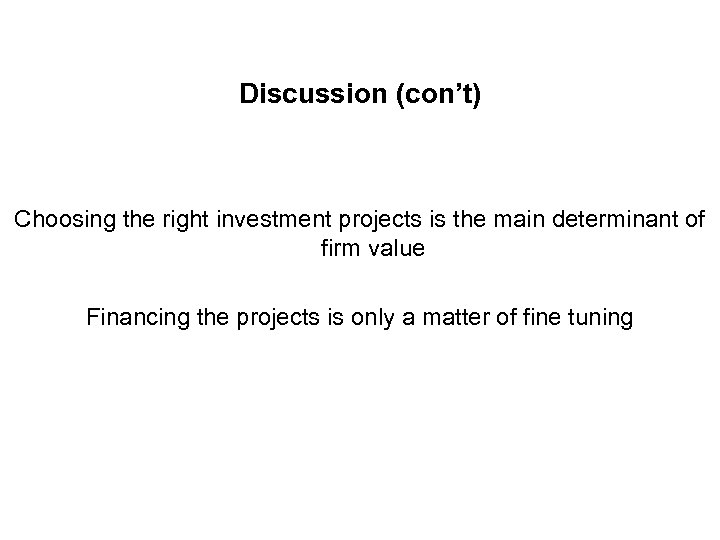Discussion (con’t) Choosing the right investment projects is the main determinant of firm value Financing the projects is only a matter of fine tuning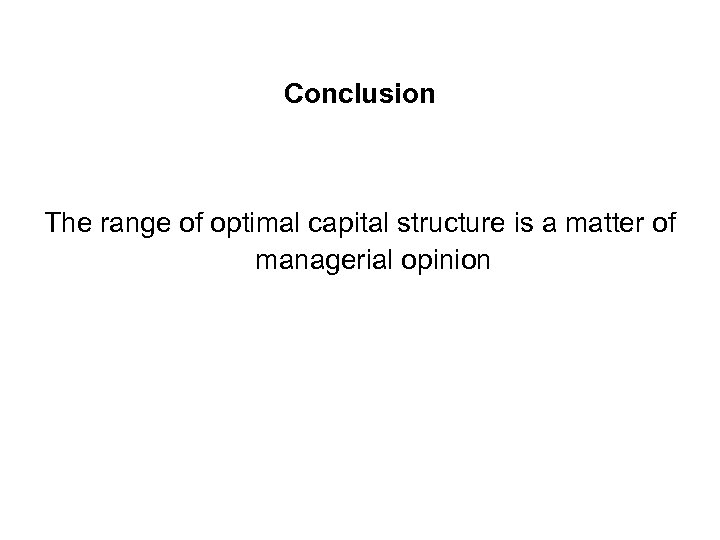Conclusion The range of optimal capital structure is a matter of managerial opinion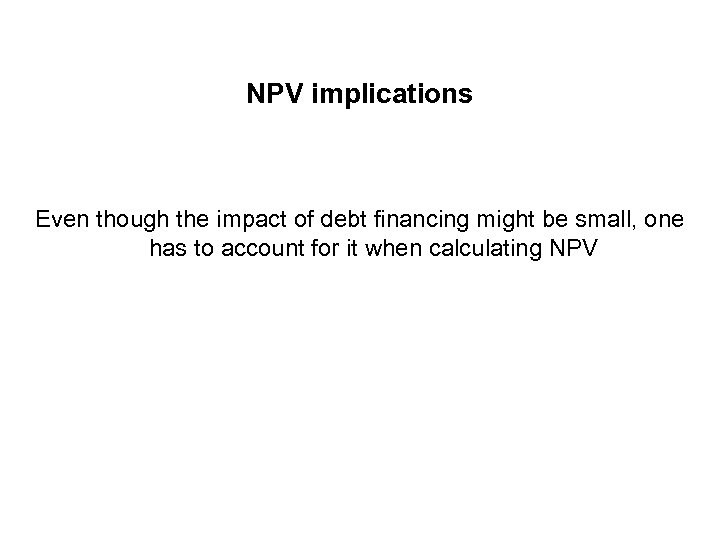NPV implications Even though the impact of debt financing might be small, one has to account for it when calculating NPV